﻿ Motions of Planets and Satellites | Aerospace Engineering

## Motions of Planets and Satellites

### Orbit Meccanics:

Through a lifelong study of the motions of bodies in the solar system, Johannes Kepler (1571-1630) was able to derive three basic laws known as Kepler’s laws of planetary motion. Using the data compiled by his mentor Tycho Brahe (1546-1601), Kepler found the following regularities after years of laborious calculations:

1.  All planets move in elliptical orbits with the sun at one focus.
2.  A line joining any planet to the sun sweeps out equal areas in equal times.
3.  The square of the period of any planet about the sun is proportional to the cube of the planet’s mean distance from the sun.

These laws can be deduced from Newton’s laws of motion and law of universal gravitation. Indeed, Newton used Kepler’s work as basic information in the formulation of his gravitational theory.

As Kepler pointed out, all planets move in elliptical orbits, however, we can learn much about planetary motion by considering the special case of circular orbits. We shall neglect the forces between planets, considering only a planet’s interaction with the sun. These considerations apply equally well to the motion of a satellite about a planet.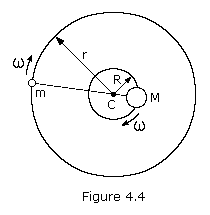Let’s examine the case of two bodies of masses M and m moving in circular orbits under the influence of each other’s gravitational attraction. The center of mass of this system of two bodies lies along the line joining them at a point C such that mr = MR. The large body of mass M moves in an orbit of constant radius R and the small body of mass m in an orbit of constant radius r, both having the same angular velocity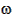. For this to happen, the gravitational force acting on each body must provide the necessary centripetal acceleration. Since these gravitational forces are a simple action-reaction pair, the centripetal forces must be equal but opposite in direction. That is, m2r must equal M2R. The specific requirement, then, is that the gravitational force acting on either body must equal the centripetal force needed to keep it moving in its circular orbit, that is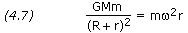If one body has a much greater mass than the other, as is the case of the sun and a planet or the Earth and a satellite, its distance from the center of mass is much smaller than that of the other body. If we assume that m is negligible compared to M, then R is negligible compared to r. Thus, equation (4.7) then becomes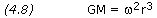If we express the angular velocity in terms of the period of revolution,= 2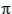/P, we obtain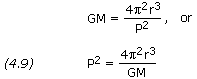where P is the period of revolution. This is a basic equation of planetary and satellite motion. It also holds for elliptical orbits if we define r to be the semi-major axis (a) of the orbit.

A significant consequence of this equation is that it predicts Kepler’s third law of planetary motion, that is P2~r3.

———————————————————————————————————————————————-

```PROBLEM 1

Calculate the period of revolution of a the satellite

SOLUTION:

Given:  r = 6,578,140 m

Equation (4.9),

P2 = 4 ×2 × r3 / GM

P = SQRT[ 4 ×2 × r3 / GM ]
P = SQRT[ 4 ×2 × 6,578,1403 / 3.986005×1014 ]
P = 5,310 s```

```PROBLEM 2

Calculate the radius of orbit for a Earth satellite in a geosynchronous orbit,
where the Earth's rotational period is 86,164.1 seconds.

SOLUTION:

Given:  P = 86,164.1 s

Equation (4.9),

P2 = 4 ×2 × r3 / GM

r = [ P2 × GM / (4 ×2) ]1/3
r = [ 86,164.12 × 3.986005×1014 / (4 ×2) ]1/3
r = 42,164,170 m```

———————————————————————————————————————————————-

 In celestial mechanics where we are dealing with planetary or stellar sized bodies, it is often the case that the mass of the secondary body is significant in relation to the mass of the primary, as with the Moon and Earth. In this case the size of the secondary cannot be ignored. The distance R is no longer negligible compared to r and, therefore, must be carried through the derivation. Equation (4.9) becomes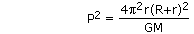More commonly the equation is written in the equivalent form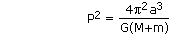where a is the semi-major axis. The semi-major axis used in astronomy is always the primary-to-secondary distance, or the geocentric semi-major axis. For example, the Moon’s mean geocentric distance from Earth (a) is 384,403 kilometers. On the other hand, the Moon’s distance from the barycenter (r) is 379,732 km, with Earth’s counter-orbit (R) taking up the difference of 4,671 km.

Kepler’s second law of planetary motion must, of course, hold true for circular orbits. In such orbits bothand r are constant so that equal areas are swept out in equal times by the line joining a planet and the sun. For elliptical orbits, however, bothand r will vary with time. Let’s now consider this case.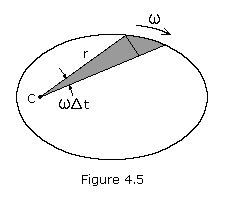Figure 4.5 shows a particle revolving around C along some arbitrary path. The area swept out by the radius vector in a short time intervalt is shown shaded. This area, neglecting the small triangular region at the end, is one-half the base times the height or approximately r(rt)/2. This expression becomes more exact ast approaches zero, i.e. the small triangle goes to zero more rapidly than the large one. The rate at which area is being swept out instantaneously is therefore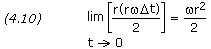For any given body moving under the influence of a central force, the valuer2 is constant.

Let’s now consider two points P1 and P2 in an orbit with radii r1 and r2, and velocities v1 and v2. Since the velocity is always tangent to the path, it can be seen that ifis the angle between r and v, then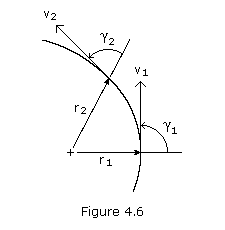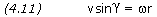where vsinis the transverse component of v. Multiplying through by r, we have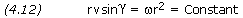or, for two points P1 and P2 on the orbital pathNote that at periapsis and apoapsis,= 90 degrees. Thus, letting P1 and P2 be these two points we get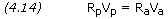Let’s now look at the energy of the above particle at points P1 and P2Conservation of energy states that the sum of the kinetic energy and the potential energy of a particle remains constant. The kinetic energy T of a particle is given by mv2/2 while the potential energy of gravity V is calculated by the equation -GMm/r. Applying conservation of energy we have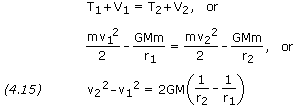From equations (4.14) and (4.15) we obtain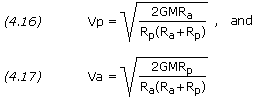Rearranging terms we get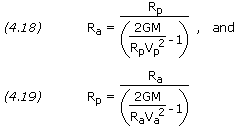———————————————————————————————————————————————-

```PROBLEM 3

An artificial Earth satellite is in an elliptical orbit which brings it to
an altitude of 250 km at perigee and out to an altitude of 500 km at
apogee.  Calculate the velocity of the satellite at both perigee and apogee.

SOLUTION,

Given:  Rp = (6,378.14 + 250) × 1,000 = 6,628,140 m
Ra = (6,378.14 + 500) × 1,000 = 6,878,140 m

Equations (4.16) and (4.17),

Vp = SQRT[ 2 × GM × Ra / (Rp × (Ra + Rp)) ]
Vp = SQRT[ 2 × 3.986005×1014 × 6,878,140 / (6,628,140 ×
(6,878,140 + 6,628,140)) ]
Vp = 7,826 m/s

Va = SQRT[ 2 × GM × Rp / (Ra × (Ra + Rp)) ]
Va = SQRT[ 2 × 3.986005×1014 × 6,628,140 / (6,878,140 ×
(6,878,140 + 6,628,140)) ]
Va = 7,542 m/s```

```PROBLEM 4

A satellite in Earth orbit passes through its perigee point
at an altitude of 200 km above the Earth's surface and at a
velocity of 7,850 m/s.  Calculate the apogee altitude of the
satellite.

SOLUTION,

Given:  Rp = (6,378.14 + 200) × 1,000 = 6,578,140 m
Vp = 7,850 m/s

Equation (4.18),

Ra = Rp / [2 × GM / (Rp × Vp2) - 1]
Ra = 6,578,140/[2×3.986005×1014/(6,578,140×7,8502)-1]
Ra = 6,805,140 m

Altitude @ apogee = 6,805,140 / 1,000 - 6,378.14 = 427.0 km```

———————————————————————————————————————————————-

The eccentricity e of an orbit is given by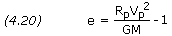———————————————————————————————————————————————-

```PROBLEM 5

Calculate the eccentricity of the orbit for the satellite in problem 4.

SOLUTION,

Given:  Rp = 6,578,140 m
Vp = 7,850 m/s

Equation (4.20),

e = Rp × Vp2 / GM - 1
e = 6,578,140 × 7,8502 / 3.986005×1014 - 1
e = 0.01696```

———————————————————————————————————————————————-

If the semi-major axis a and the eccentricity e of an orbit are known, then the periapsis and apoapsis distances can be calculated by———————————————————————————————————————————————-

```PROBLEM 6

A satellite in Earth orbit has a semi-major axis of 6,700 km and an
eccentricity of 0.01. Calculate the satellite's altitude at both
perigee and apogee.

SOLUTION,

Given:  a = 6,700 km
e = 0.01

Equation (4.21) and (4.22),

Rp = a × (1 - e)
Rp = 6,700 × (1 - .01)
Rp = 6,633 km

Altitude @ perigee = 6,633 - 6,378.14 = 254.9 km

Ra = a × (1 + e)
Ra = 6,700 × (1 + .01)
Ra = 6,767 km

Altitude @ apogee = 6,767 - 6,378.14 = 388.9 km```

##### About Aerospace Engineering - Training
Aerospace Engineering-Training is an online learning platform. It is aimed at professional adults.
This entry was posted in Orbital Mechanics and tagged , , , , , , , , , , , , , , , , , , , , , , , , , , , . Bookmark the permalink.